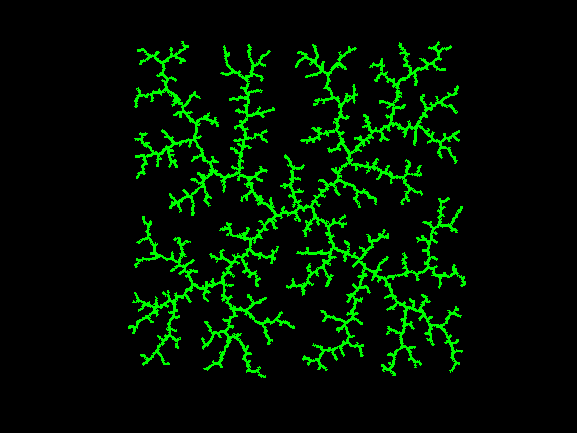# Rapidly-exploring random tree (RRT)Juan Villacrés on 17 Oct 2021
• 38
• 90
• 1
• 0
• 232
% Graphic inspired by the "BuildRRT" function of:
% LaValle, Steven M. (October 1998). "Rapidly-exploring random trees: A new tool for path planning"
% The origin is (0,0)
figure('Color','k')
l=5000;
a=zeros(l,2);
for i=2:l
b=200*(rand(1,2)-.5);
[~,w]=min(sum((a-b).^2,2));
n=a(w,:);
k=atan2(b(2)-n(2),b(1)-n(1));
c=[n(1)+cos(k) n(2)+sin(k)];
a(i,:)=c;
plot([n(1) c(1)],[n(2) c(2)],'g','Linew',2)
hold on
end
axis off square